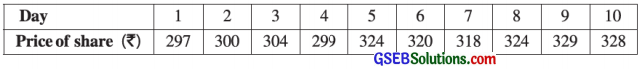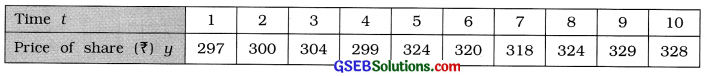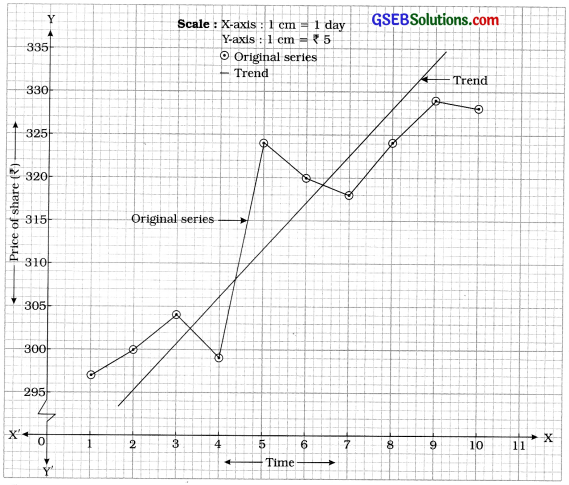# GSEB Solutions Class 12 Statistics Part 1 Chapter 4 Time Series Ex 4.1

Gujarat Board Statistics Class 12 GSEB Solutions Part 1 Chapter 4 Time Series Ex 4.1 Textbook Exercise Questions and Answers.

## Gujarat Board Textbook Solutions Class 12 Statistics Part 1 Chapter 4 Time Series Ex 4.1

Question 1.
The information about the capacity (in lakh tons) to load ships at a port each year is given below. Find the linear trend using graphical method.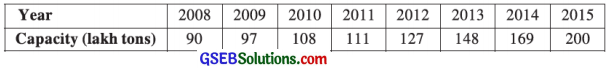Here, unit of time is year; y = capacity; t assumes 2008, 2009, …………. 2015. Taking origin at t = 2007, the given time series is written as shown in the following table: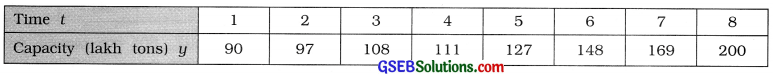Taking t on X-axis and y on Y-axis, the points (1, 90), (2, 97), …………. (8, 200) are plotted on the graph paper. Joining these points serially by straight lines, the curve of the given time series is obtained as follows: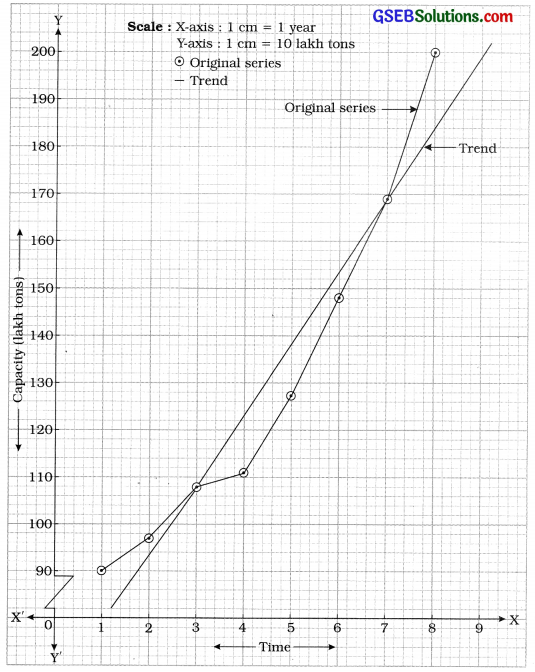The curve passing through close to most of the points of the curve of original. Series is showing the trend of given time series.Question 2.
The number of tourists (in thousand) visiting a certain tourist place is as follows. Find the trend using a suitable graph.Here, the unit of time is year; y = No. of tourists; t assumes 2010, 2011, ……………. 2016. Taking origin at t = 2009 the given time series is written as shown in the following table: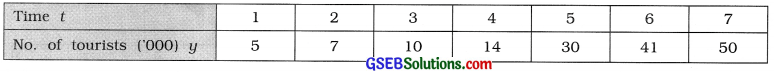Taking t on X-axis and y on Y-axis, the points (1, 5), (2, 7), ………., (7, 50) are plotted on the graph paper. Joining these points serially by straight lines, the curve of the given time series is obtained as follows: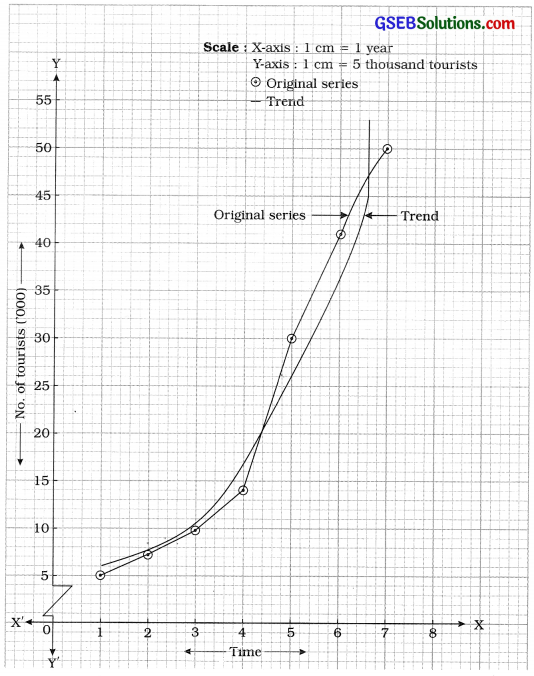The curve passing through close to most of the points of the curve of original series is showing trend of the given time series.

Question 3.
The data regarding number of girls (yt) per 1000 boys in the age group 0-6 years of a state are given in the following table. Obtain the linear trend using graphical method.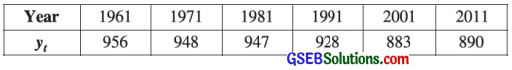Here, the unit time of is year; y = yt = No. of girls per 1000 boys; t assumes 1961, 1971, …………. 2011. Taking origin at t = 1951 and scale to 10 years the given time series is written as shown in the following table: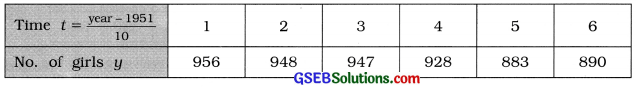Taking t on X-axis and y on Y-axis, the points (1, 956), (2, 948), …….., (6, 890) are plotted on the graph paper. Joining these points serially by straight lines, the curve of the given time series is obtained as follows ;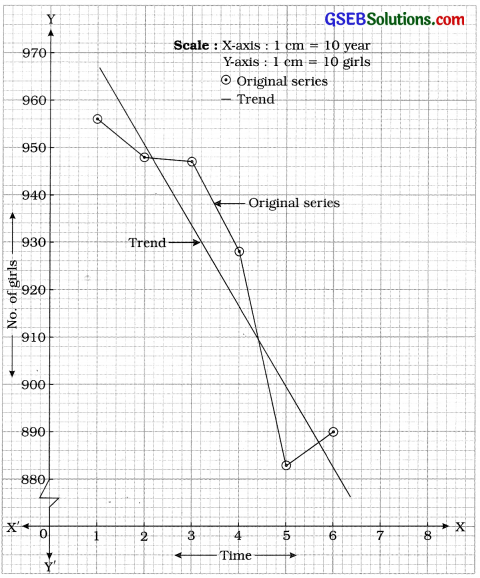The curve passing through close to most of the points of the curve of original series is showing the trend of the given time series.Question 4.
The data about the closing prices of shares of a company for 10 days are given in the following table. Obtain the trend using graphical method.